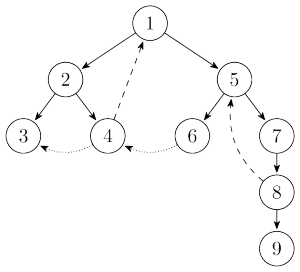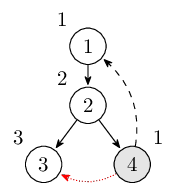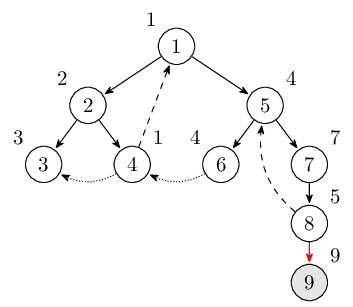Tarjan 算法是由 Robert Tarjan 提出的用于寻找有向图的强连通分量的算法。它可以在$\mathcal O(|V|+|E|)$的时间内得出结果。

Tarjan 算法主要是利用 DFS 来寻找强连通分量的。在介绍该算法之前，我们先来介绍一下搜索树。先前那个有向图的搜索树是这样的：Tarjan 算法主要是在 DFS 的过程中维护了一些信息：dfn、low 和一个栈。

• 栈里的元素表示的是当前已经访问过但是没有被归类到任一强连通分量的结点。
• dfn[u] 表示结点 u 在 DFS 中第一次搜索到的次序，通常被叫做时间戳。
• low[u] 稍微有些复杂，它表示从 u 或者以 u 为根的子树中的结点，再通过一条反祖边或者横叉边可以到达的时间戳最小的结点 v 的时间戳，并且要求 v 有一些额外的性质：v 还要能够到达 u。显然通过反祖边到达的结点 v 满足 low 的性质，但是通过横叉边到达的却不一定。

1. 结点 u 通过树边到达结点 v

$\begin{equation*} \text{low}[u] = \min(\text{low}[u], \text{low}[v]) \end{equation*}$
2. 结点 u 通过反祖边到达结点 v，或者通过横叉边到达结点 v 并且满足 low 定义中 v 的性质

$\begin{equation*} \text{low}[u] = \min(\text{low}[u], \text{dfn}[v]) \end{equation*}$